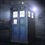# $A\ very\ simple,yet\ elegant\ NT\ proof(corrected)$

$It\ is\ a\ well\ known\ fact\ that\ if\ in\ a\ fraction,the\ denominator\ is\ of\ the\ \\ form\ 2^{m}*5^{n}\ where\ m\ and\ n\ are\ non-negative\ integers\ the\ fraction\ \\ has\ a\ \\ terminating\ decimal.$ $Here\ is\ the\ proof:$ $Let\ us\ say\ that\ there\ is\ a\ fraction\ in\ which\ the\ numerator\ is\ N\ and\ \\ the\ denominator\ be\ 2^{m}*5^{n}\ where\ m+n=x$ $The\ fraction\ can\ be\ written\ as:\\ \dfrac{N}{2^{x-n}*5^{x-m}}$ $Multiplying\ by\ \dfrac{2^{n}}{2^{n}}\ and\ then\ by\ \dfrac{5^{m}}{5^{m}}\\ we\ have\ a\ fraction\ which\ has\ a\ new\ numerator,let\ us\ call\ it\ A.$\ $The\ new\ denominator\ is\ 10^{x}\\ Thus,the\ new\ fraction\ is\ \dfrac{A}{10^{x}}.$\ $Which,obviously,has\ a\ terminating\ decimal\ expansion.$6 years, 10 months ago

This discussion board is a place to discuss our Daily Challenges and the math and science related to those challenges. Explanations are more than just a solution — they should explain the steps and thinking strategies that you used to obtain the solution. Comments should further the discussion of math and science.

When posting on Brilliant:

• Use the emojis to react to an explanation, whether you're congratulating a job well done , or just really confused .
• Ask specific questions about the challenge or the steps in somebody's explanation. Well-posed questions can add a lot to the discussion, but posting "I don't understand!" doesn't help anyone.
• Try to contribute something new to the discussion, whether it is an extension, generalization or other idea related to the challenge.

MarkdownAppears as
*italics* or _italics_ italics
**bold** or __bold__ bold
- bulleted- list
• bulleted
• list
1. numbered2. list
1. numbered
2. list
Note: you must add a full line of space before and after lists for them to show up correctly
paragraph 1paragraph 2

paragraph 1

paragraph 2

[example link](https://brilliant.org)example link
> This is a quote
This is a quote
    # I indented these lines
# 4 spaces, and now they show
# up as a code block.

print "hello world"
# I indented these lines
# 4 spaces, and now they show
# up as a code block.

print "hello world"
MathAppears as
Remember to wrap math in $$ ... $$ or $ ... $ to ensure proper formatting.
2 \times 3 $2 \times 3$
2^{34} $2^{34}$
a_{i-1} $a_{i-1}$
\frac{2}{3} $\frac{2}{3}$
\sqrt{2} $\sqrt{2}$
\sum_{i=1}^3 $\sum_{i=1}^3$
\sin \theta $\sin \theta$
\boxed{123} $\boxed{123}$

Sort by:

- 6 years, 10 months ago

WOW @Krishna Ar, this is called popularity!

- 6 years, 10 months ago

hehe

- 6 years, 10 months ago

-_-

- 6 years, 10 months ago

(y)

- 6 years, 10 months ago

Nice note, Adarsh. You may also like to add the number of decimal digits after a number of such a form terminates. It's closely related to your proof. Also, you don't need to latex the whole thing. If you are using a latex editor, then there's no need. If you want to learn it, you can easily do so here. Thanks.

- 6 years, 10 months ago

thanx.But I recently learned LATEX and now I can't get enough of it.

- 6 years, 10 months ago

BTW which class r u in?

- 6 years, 10 months ago

I'm in class 10.

- 6 years, 10 months ago

oh,ok.

- 6 years, 10 months ago

I don't understand "I can't get enough of it", you can always learn it. It's really easy to learn.

- 6 years, 10 months ago

"I can't get enough of it" means now I can't resist writing it.It is an idiom,I think.

- 6 years, 10 months ago

Do you have the proof of the property of recurring decimal expansions that they repeat for n-1 digits in a fraction 1/n?

- 6 years, 10 months ago

That's not necessary. It is only true in case of cyclic numbers. I was referring to the number of after-decimal digits after $\frac{x}{2^m5^n}$ terminates. It's the larger of $m$ and $n$.

- 6 years, 10 months ago

hmmm

- 6 years, 10 months ago

It is a very simple proof which was in my RD SHARMA but I thought it too elegant not to share it with you guys.

- 6 years, 10 months ago

expect the unexpected !

- 5 years, 8 months ago